### Home > INT2 > Chapter 12 > Lesson 12.1.1 > Problem12-9

12-9.
1. Use circle relationships to solve for the variables in each of the diagrams below. Homework Help ✎

2.  a. AB and CD intersect at E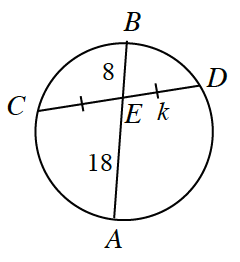b. Point C is the center.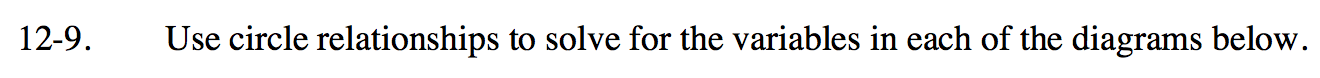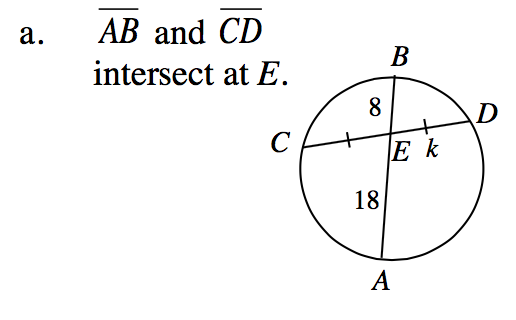(CE)(ED) = (AE)(EB)

(k)(k) = (18)(8)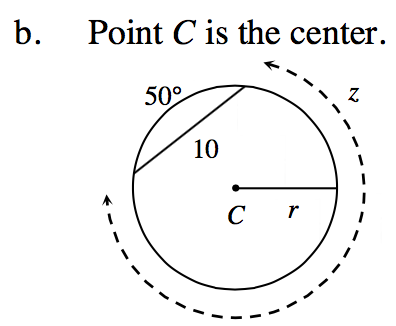Draw a perpendicular bisector on the chord to the center
of the circle, and then create two triangles.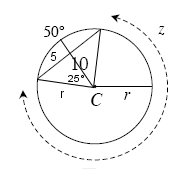$\text{sin}25^\circ = \frac{5}{r}$

r ≈ 11.83